## 5.37. atleast_nvalue

Origin
Constraint

$\mathrm{𝚊𝚝𝚕𝚎𝚊𝚜𝚝}_\mathrm{𝚗𝚟𝚊𝚕𝚞𝚎}\left(\mathrm{𝙽𝚅𝙰𝙻},\mathrm{𝚅𝙰𝚁𝙸𝙰𝙱𝙻𝙴𝚂}\right)$

Synonym

$𝚔_\mathrm{𝚍𝚒𝚏𝚏}$.

Arguments
 $\mathrm{𝙽𝚅𝙰𝙻}$ $\mathrm{𝚍𝚟𝚊𝚛}$ $\mathrm{𝚅𝙰𝚁𝙸𝙰𝙱𝙻𝙴𝚂}$ $\mathrm{𝚌𝚘𝚕𝚕𝚎𝚌𝚝𝚒𝚘𝚗}\left(\mathrm{𝚟𝚊𝚛}-\mathrm{𝚍𝚟𝚊𝚛}\right)$
Restrictions
 $\mathrm{𝚛𝚎𝚚𝚞𝚒𝚛𝚎𝚍}$$\left(\mathrm{𝚅𝙰𝚁𝙸𝙰𝙱𝙻𝙴𝚂},\mathrm{𝚟𝚊𝚛}\right)$ $\mathrm{𝙽𝚅𝙰𝙻}\ge 0$ $\mathrm{𝙽𝚅𝙰𝙻}\le |\mathrm{𝚅𝙰𝚁𝙸𝙰𝙱𝙻𝙴𝚂}|$ $\mathrm{𝙽𝚅𝙰𝙻}\le$$\mathrm{𝚛𝚊𝚗𝚐𝚎}$$\left(\mathrm{𝚅𝙰𝚁𝙸𝙰𝙱𝙻𝙴𝚂}.\mathrm{𝚟𝚊𝚛}\right)$
Purpose

The number of distinct values taken by the variables of the collection $\mathrm{𝚅𝙰𝚁𝙸𝙰𝙱𝙻𝙴𝚂}$ is greater than or equal to $\mathrm{𝙽𝚅𝙰𝙻}$.

Example
 $\left(2,〈3,1,7,1,6〉\right)$ $\left(4,〈3,1,7,1,6〉\right)$ $\left(5,〈3,1,7,0,6〉\right)$

The first $\mathrm{𝚊𝚝𝚕𝚎𝚊𝚜𝚝}_\mathrm{𝚗𝚟𝚊𝚕𝚞𝚎}$ constraint holds since the collection $〈3,1,7,1,6〉$ involves at least 2 distinct values (i.e., in fact 4 distinct values).

Typical
 $\mathrm{𝙽𝚅𝙰𝙻}>0$ $\mathrm{𝙽𝚅𝙰𝙻}<|\mathrm{𝚅𝙰𝚁𝙸𝙰𝙱𝙻𝙴𝚂}|$ $\mathrm{𝙽𝚅𝙰𝙻}<$$\mathrm{𝚛𝚊𝚗𝚐𝚎}$$\left(\mathrm{𝚅𝙰𝚁𝙸𝙰𝙱𝙻𝙴𝚂}.\mathrm{𝚟𝚊𝚛}\right)$ $|\mathrm{𝚅𝙰𝚁𝙸𝙰𝙱𝙻𝙴𝚂}|>1$
Symmetries
• $\mathrm{𝙽𝚅𝙰𝙻}$ can be decreased to any value $\ge 0$.

• Items of $\mathrm{𝚅𝙰𝚁𝙸𝙰𝙱𝙻𝙴𝚂}$ are permutable.

• All occurrences of two distinct values of $\mathrm{𝚅𝙰𝚁𝙸𝙰𝙱𝙻𝙴𝚂}.\mathrm{𝚟𝚊𝚛}$ can be swapped; all occurrences of a value of $\mathrm{𝚅𝙰𝚁𝙸𝙰𝙱𝙻𝙴𝚂}.\mathrm{𝚟𝚊𝚛}$ can be renamed to any unused value.

Arg. properties

Extensible wrt. $\mathrm{𝚅𝙰𝚁𝙸𝙰𝙱𝙻𝙴𝚂}$.

Remark

The $\mathrm{𝚊𝚝𝚕𝚎𝚊𝚜𝚝}_\mathrm{𝚗𝚟𝚊𝚕𝚞𝚎}$ constraint was first introduced by J.-C. Régin under the name $𝚔_\mathrm{𝚍𝚒𝚏𝚏}$ in [Regin95]. Later on the $\mathrm{𝚊𝚝𝚕𝚎𝚊𝚜𝚝}_\mathrm{𝚗𝚟𝚊𝚕𝚞𝚎}$ constraint was introduced together with the $\mathrm{𝚊𝚝𝚖𝚘𝚜𝚝}_\mathrm{𝚗𝚟𝚊𝚕𝚞𝚎}$ constraint by C. Bessière et al. in an article [BessiereHebrardHnichKiziltanWalsh05] providing filtering algorithms for the $\mathrm{𝚗𝚟𝚊𝚕𝚞𝚎}$ constraint.

Algorithm

[BessiereHebrardHnichKiziltanWalsh05] provides a sketch of a filtering algorithm enforcing arc-consistency for the $\mathrm{𝚊𝚝𝚕𝚎𝚊𝚜𝚝}_\mathrm{𝚗𝚟𝚊𝚕𝚞𝚎}$ constraint. This algorithm is based on the maximal matching in a bipartite graph.

Counting
 Length ($n$) 2 3 4 5 6 7 8 Solutions 24 212 2470 35682 614600 12286024 279472266

Number of solutions for $\mathrm{𝚊𝚝𝚕𝚎𝚊𝚜𝚝}_\mathrm{𝚗𝚟𝚊𝚕𝚞𝚎}$: domains $0..n$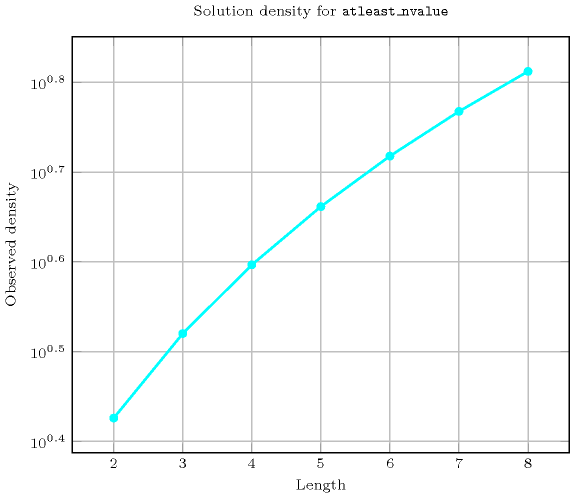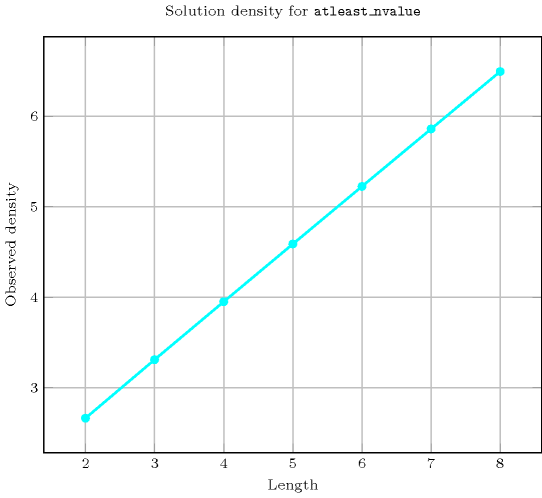Length ($n$)2345678
Total2421224703568261460012286024279472266
 Parameter value

09646257776117649209715243046721
19646257776117649209715243046721
26606207770117642209714443046712
3-244807320116340209361643037568
4--120432097440199248042550704
5---72042840140448037406880
6----504046368021530880
7-----403205443200
8------362880

Solution count for $\mathrm{𝚊𝚝𝚕𝚎𝚊𝚜𝚝}_\mathrm{𝚗𝚟𝚊𝚕𝚞𝚎}$: domains $0..n$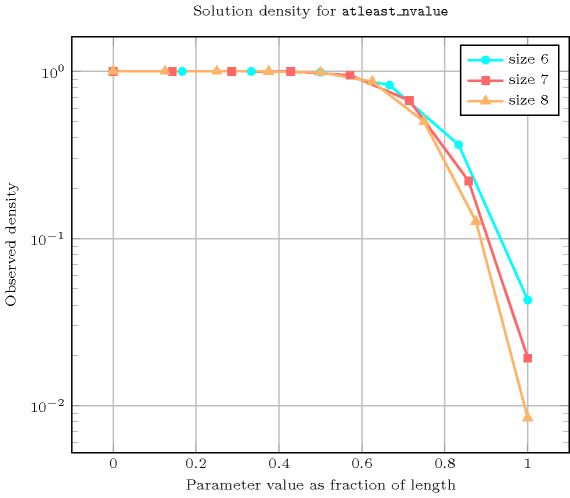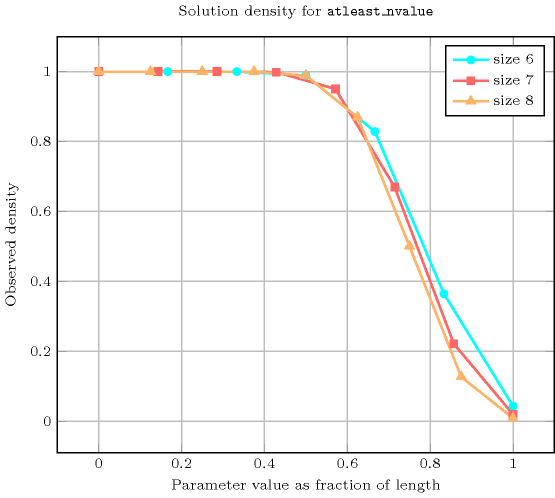implied by: $\mathrm{𝚊𝚗𝚍}$, $\mathrm{𝚎𝚚𝚞𝚒𝚟𝚊𝚕𝚎𝚗𝚝}$, $\mathrm{𝚒𝚖𝚙𝚕𝚢}$, $\mathrm{𝚗𝚊𝚗𝚍}$, $\mathrm{𝚗𝚘𝚛}$, $\mathrm{𝚗𝚟𝚊𝚕𝚞𝚎}$ ($\ge$ $\mathrm{𝙽𝚅𝙰𝙻}$ replaced by $=$ $\mathrm{𝙽𝚅𝙰𝙻}$), $\mathrm{𝚗𝚟𝚒𝚜𝚒𝚋𝚕𝚎}_\mathrm{𝚏𝚛𝚘𝚖}_\mathrm{𝚎𝚗𝚍}$, $\mathrm{𝚗𝚟𝚒𝚜𝚒𝚋𝚕𝚎}_\mathrm{𝚏𝚛𝚘𝚖}_\mathrm{𝚜𝚝𝚊𝚛𝚝}$, $\mathrm{𝚘𝚛}$, $\mathrm{𝚜𝚒𝚣𝚎}_\mathrm{𝚖𝚊𝚡}_\mathrm{𝚜𝚎𝚚}_\mathrm{𝚊𝚕𝚕𝚍𝚒𝚏𝚏𝚎𝚛𝚎𝚗𝚝}$, $\mathrm{𝚜𝚒𝚣𝚎}_\mathrm{𝚖𝚊𝚡}_\mathrm{𝚜𝚝𝚊𝚛𝚝𝚒𝚗𝚐}_\mathrm{𝚜𝚎𝚚}_\mathrm{𝚊𝚕𝚕𝚍𝚒𝚏𝚏𝚎𝚛𝚎𝚗𝚝}$, $\mathrm{𝚡𝚘𝚛}$.

Keywords
Arc input(s)

$\mathrm{𝚅𝙰𝚁𝙸𝙰𝙱𝙻𝙴𝚂}$

Arc generator
$\mathrm{𝐶𝐿𝐼𝑄𝑈𝐸}$$↦\mathrm{𝚌𝚘𝚕𝚕𝚎𝚌𝚝𝚒𝚘𝚗}\left(\mathrm{𝚟𝚊𝚛𝚒𝚊𝚋𝚕𝚎𝚜}\mathtt{1},\mathrm{𝚟𝚊𝚛𝚒𝚊𝚋𝚕𝚎𝚜}\mathtt{2}\right)$

Arc arity
Arc constraint(s)
$\mathrm{𝚟𝚊𝚛𝚒𝚊𝚋𝚕𝚎𝚜}\mathtt{1}.\mathrm{𝚟𝚊𝚛}=\mathrm{𝚟𝚊𝚛𝚒𝚊𝚋𝚕𝚎𝚜}\mathtt{2}.\mathrm{𝚟𝚊𝚛}$
Graph property(ies)
$\mathrm{𝐍𝐒𝐂𝐂}$$\ge \mathrm{𝙽𝚅𝙰𝙻}$

Graph class
$\mathrm{𝙴𝚀𝚄𝙸𝚅𝙰𝙻𝙴𝙽𝙲𝙴}$

Graph model

Parts (A) and (B) of Figure 5.37.1 respectively show the initial and final graph associated with the first example of the Example slot. Since we use the $\mathrm{𝐍𝐒𝐂𝐂}$ graph property we show the different strongly connected components of the final graph. Each strongly connected component corresponds to a specific value that is assigned to some variables of the $\mathrm{𝚅𝙰𝚁𝙸𝙰𝙱𝙻𝙴𝚂}$ collection. The 4 following values 1, 3, 6 and 7 are used by the variables of the $\mathrm{𝚅𝙰𝚁𝙸𝙰𝙱𝙻𝙴𝚂}$ collection.

##### Figure 5.37.1. Initial and final graph of the $\mathrm{𝚊𝚝𝚕𝚎𝚊𝚜𝚝}_\mathrm{𝚗𝚟𝚊𝚕𝚞𝚎}$ constraint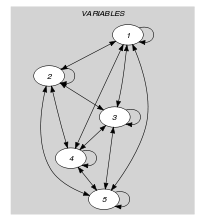(a)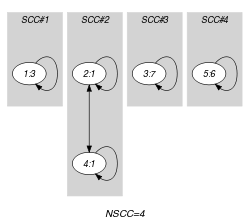(b)